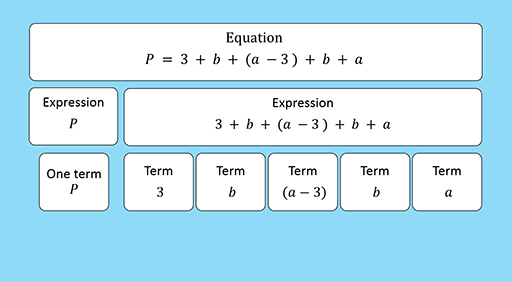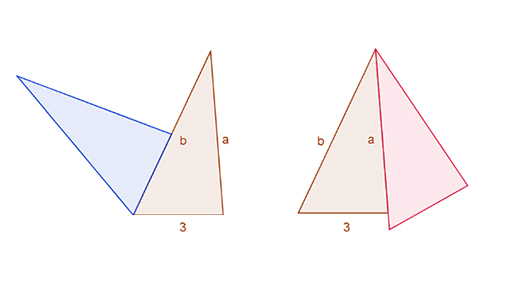Education & Development

### Become an OU studentTeaching mathematics

Start this free course now. Just create an account and sign in. Enrol and complete the course for a free statement of participation or digital badge if available.

# 1.2 Expressions and terms

An algebraic expression is a calculation, written in standard arithmetic notation, where one or more numbers has been replaced with a symbol. If an expression involves addition or subtraction then the parts that are added or subtracted are called ‘terms’. The video below shows the parts of an equation which are algebraic expressions and then shows how the expressions are made up of algebraic terms.Video 1 Expressions and terms
Interactive feature not available in single page view (see it in standard view).

## Differences between talking in mathematical sentences and writing algebraic expressions

It is useful for teachers to compare spoken explanations and algebraic expressions.

The explanations in Activity 2 above are written in informal language, not in whole sentences, but as learners might say them.

Learners typically explain ideas in several short sentences, each with one operation. The sentences may follow the order of their thinking (for example, the first instruction will involve ‘adding’ since the aim is a perimeter). This is unlikely to match the order of the terms in the conventional expression.

When we read, we read from left to right. When we talk, our sentences usually start with the main subject. However, in numerical or algebraic expressions, the first term is not the most important one, nor is it always the unknown one, as in the examples above. The order in which you do operations is not necessarily the same order as you read and write the symbols.

Note how learners typically use ‘pointing’ words such as ‘this’, ‘that’ or ‘there’ to indicate a quantity that they want to refer to, but cannot name it without losing the thread of their thinking. In the classroom, they may also point to the diagram or one of the sides while they are talking. Gestures can be an important way of signalling their awareness of an unknown quantity. In an expression, this quantity might appear in brackets, or as a more complex term.

These are steps towards algebraic thinking. Learners have paid attention to an unknown quantity and are communicating about it.

How would you as a teacher build on the learner’s talk, and rephrase each explanation to make a more precise mathematical sentence? Think about using words such as ‘difference’ and ‘less than’.

(We say more precise here since it is not always easy to express mathematical relationships concisely and precisely in words – that is why algebra was invented.)

## Activity 3 Reflecting

Timing: Allow 5 minutes

Look back in the diagram in Activity 2, how would the task be different if a and b were replaced by specific numbers? How could you work with learners to keep the focus on mathematical sentences?

### Discussion

When given specific numbers, learners tend to work towards closed answers consisting of a single term. For example, if the triangles were labelled with sides 3, 5 (for a) and 7 (for b), they would add up as they went around the perimeter and get the answer 24. You would need to ask learners to write down mathematical sentences to keep the focus on the instructions, not the answer, such as writing down 10 + (5 – 3) + 5 + 7 = 24.

When there are specific numbers, learners find it easier to identify a number that they can use for the ‘leftover bit’.

For a general side a, the difference is written a – 3. It cannot be simplified further but you can write it instead of a number and give the instruction to add it to the other sides. Learners often like to use brackets to show that this is an expression that is being treated as a single object.

One strategy for helping those who are stuck on this part is to say ‘Suppose a was 5, how long is that bit?’ There is then a danger that they spot that the difference is 2 using only their knowledge of the specific numbers 5 and 3. You can help them pay attention to the general structure by recapping that the difference is 2 because 5 – 3 is 2, so the important operation is subtraction.

## Activity 4 Varying the problem

Timing: Allow 10 minutes

What happens to the perimeter if you combine the two congruent triangles into the new shapes in Figure 2?

Use the mathematical extensions for greater depth:

What other shapes and perimeters can you find with these triangles?

Could you use these triangles to make a shape with perimeter 2a + 2b + 3?Figure 2 Four triangles

### Discussion

If you join the two triangles together with the whole side of one triangle set against the same length side of the other triangle, you can arrange the triangles into many shapes, including a rectangle, a parallelogram and a kite. Whatever the shape, the perimeter will always simplify to an expression that is double the sum of two of the sides.

If you change the rule slightly, then you can find some other perimeters. For example, they could meet just at one point, or the sides could overlap by only half their length.

## Activity 5 Is this task algebraic?

Timing: Allow 5 minutes

The beginning of this section defined algebraic thinking as involving indeterminacy, denotation and analyticity. In other words, it involves thinking about indeterminate quantities that are represented in words or symbols and then treated analytically.

Do you consider the triangle task algebraic?

### Discussion

Although the task is set in a geometric context and needs an idea of perimeter, it is algebraic.

It involves indeterminacy, as learners are working with the unknown quantities a, b and p, and these are treated analytically since they are operated on as if they were known numbers. The quantities are represented on a diagram to give a concrete basis for the reasoning. Learners also represent, or denote, them symbolically using letters and numbers and iconically using words to identify and name the quantities (‘the difference’, ‘the little bit’) or to ‘point’ to them (‘that one’). The fact that the answer cannot be reduced to one specific number or closed answer is also characteristic of algebraic thinking.